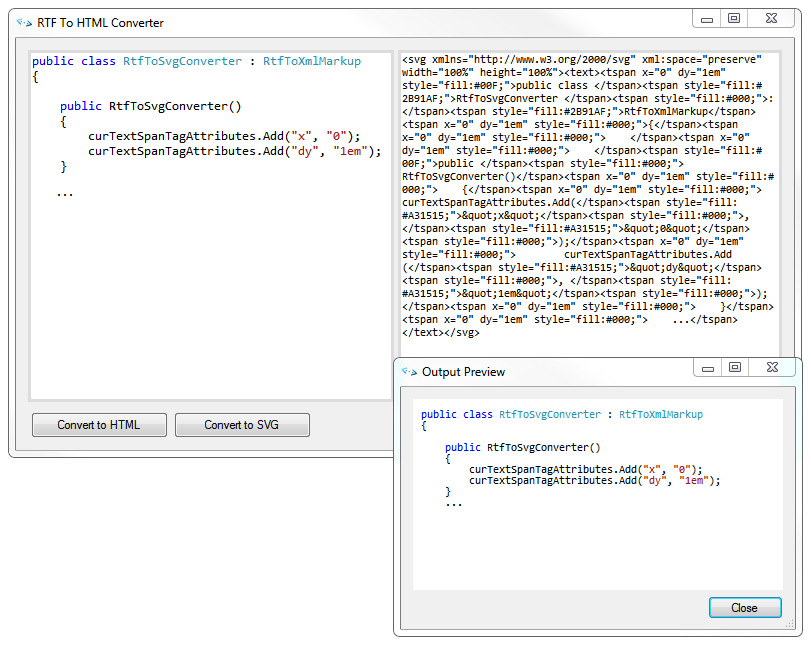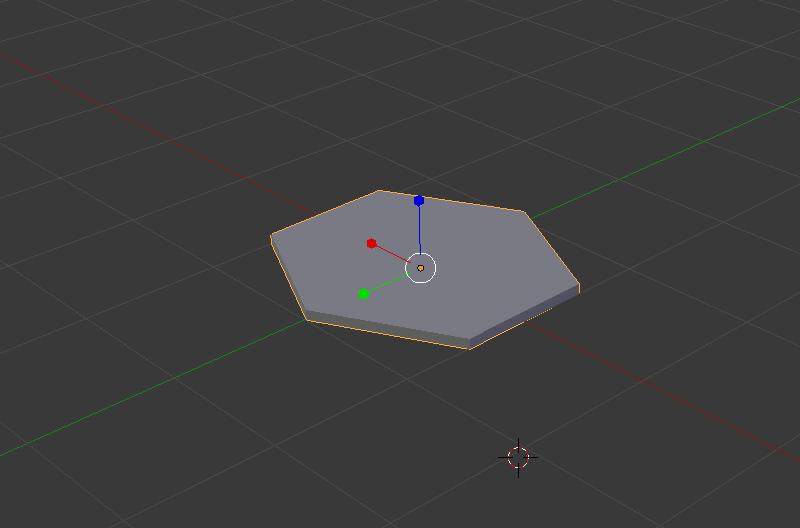## Archive for October, 2014

### Rtf2Html 1.3

I recently made a small update to Rtf2Html (the converter I wrote for converting RTF text to HTML markup):

• Support for conversion to SVG markup
• Updated preview form to use GeckoFX 29 and XULRunner 29.0.1 (the major version numbers have to match)

Note that this version requires the .NET Framework v4.0 or later.While you can put the generated SVG text on a page, that wasn’t really my motivation here; what I wanted was a way to import syntax-highlighted text (in my case, typically code) into a vector graphics application (Inkscape, Illustrator, etc.) to be placed as part of a diagram.

### Where we live

Using a number of technologies I’ve been playing around with recently, I began working on a 3D visualization of the Earth, plotting every city, creating a pointillism-styled representation of the planet. Below is the result along with an overview of how I produced the rendering.

#### Getting the data

I extracted all cities with a population of at least 100,000 people from the MySQL GeoNames database using the following query:

`SELECT `id`,`name`,`latitude`,`longitude`,`population`,`timezone`FROM geonames.citiesWHERE population >= 100000 AND feature_class = 'P';`

… and put the results into a JS array.

#### Creating a 3D model to represent each city

I created this hexagonal model in Blender, exported it to a Wavefront OBJ file, and ran the OBJ file through the Wavefront OBJ to JSON converter I wrote. Note that the model is facing the z-axis to match WebGL’s (and OpenGL’s) default camera orientation: facing down the negative z-axis.#### Convert longitude and latitude to a 3D position

Converting a geodetic longitude, latitude pair to a 3D position involves doing a LLA (Longitude Latitude Altitude) to ECEF (Earth-Centered, Earth-Fixed) transformation. The code below implements this transform, converting the longitude and latitude of every city pulled from the GeoNames database into a 3D coordinate where we can render the hexagonal representation of the city.

`function llarToWorld(lat, lon, alt, rad) {                lat = lat * (Math.PI/180.0);    lon = lon * (Math.PI/180.0);    var f = 0; //flattening    var ls = Math.atan( Math.pow((1.0 - f),2) * Math.tan(lat) ); // lambda    var x = rad * Math.cos(ls) * Math.cos(lon) + alt * Math.cos(lat) * Math.cos(lon)    var y = rad * Math.cos(ls) * Math.sin(lon) + alt * Math.cos(lat) * Math.sin(lon)    var z = rad * Math.sin(ls) + alt * Math.sin(lat)        return [x,z,-y];            }`

There are 2 items worth noting:

• The transformation (and function above) involve a 4th parameter, radius which is the radius of the ellipsoid (or sphere, in this case, as flattening=0) into which the transformation is done. I have it set as a fixed constant, as I’m primary concerned with an approximate visual representation, but the MathWorks page describes the actual computation.
• The ECEF (Earth-Centered, Earth-Fixed) coordinate system has the z-axis pointing north, not the y-axis, so the z and y values need to be swapped to produce a coordinate corresponding to WebGL’s default camera orientation. In addition, as WebGL has a right-handed coordinate system (so the default camera orientation is one where it’s pointing down the negative z-axis), the z coordinate is negated so the point doesn’t wind up behind the camera.

#### Orient all cities to face the origin

Getting each of the hexagonal models to face the origin involved a bit of math:

• Calculating the axis about which the rotation should occur by, first, computing a vector from the origin to the 3D position of the model (lookAt), and taking the cross product between lookAt and the z-axis (as we’re rotating toward the z-axis).
• Calculating the angle of rotation (the angle between the z-axis and lookAt) by computing the dot product between lookAt and the z-axis, then taking the acos of the dot product.

There’s some additional code to handle cases where points lie on the on the z-axis (where the cross product gives the zero vector) and also to return a matrix representation of the rotation.

` function lookAtOrigin(v) { // compute vector from origin var lookAt = vec3.create([v, v, -v]); vec3.normalize(lookAt); // reference axis var refAxis = vec3.create([0,0,-1]); // computate axis of rotation var rotAxis = vec3.create(lookAt); vec3.cross(rotAxis, refAxis); // compute angle of rotation var rotAngRad = Math.acos(vec3.dot(lookAt, refAxis)); // special cases... if(rotAxis == 0 && rotAxis == 0 && rotAxis == 0) { if(lookAt > 0) { rotAxis = vec3.create([1,0,0]); rotAngRad = Math.PI; } else { rotAxis = vec3.create([1,0,0]); rotAngRad = 0; } } // compute and return a matrix with the rotation var ret = mat4.identity(); mat4.rotate(ret, rotAngRad, rotAxis); return ret; }`

#### Render the scene

Using glfx, I pulled everything together, also adding a bit of code to rotate the camera and do some pseudo-lighting in the pixel shader by alpha blending colors based on depth. All the code can be found in the webgl-globe repository on bitbucket.